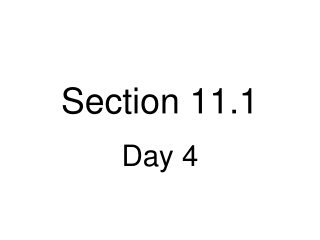DownloadDownload PresentationSection 11.1

# Section 11.1

Télécharger la présentation## Section 11.1

- - - - - - - - - - - - - - - - - - - - - - - - - - - E N D - - - - - - - - - - - - - - - - - - - - - - - - - - -
##### Presentation Transcript

1. Section 11.1 Day 4

2. Plot height vs age Which goes on x-axis and which on y-axis?

3. Plot height vs age response vs explanatory Which goes on x-axis and which on y-axis?

4. Plot height vs age response vs explanatory Which goes on x-axis and which on y-axis? x-axis: age y-axis: height

5. Formula for estimating the standard error of the slope b1.

6. Key Concept The slope b1 of the regression line variesless from sample to sample when: • Sample size is ______________ • Residuals are _______________ • Values of x are ______________

7. Key Concept The slope b1 of the regression line variesless from sample to sample when: • Sample size is larger • Residuals are _______________ • Values of x are ______________

8. Key Concept The slope b1 of the regression line variesless from sample to sample when: • Sample size is larger • Residuals are smaller • Values of x are ______________

9. Key Concept The slope b1 of the regression line variesless from sample to sample when: • Sample size is larger • Residuals are smaller • Values of x are further apart

10. Page 749, P3

11. Page 749, P3

12. Page 749, P3 Response variable y is opening day Predictor variable is swe (snow water equivalent)

13. Page 749, P3 Response variable y is opening day Predictor variable is swe (snow water equivalent) opening day = b0 + b1●swe

14. Page 749, P3 opening day = b0 + b1●swe When the amount of snow was 30 inches of swe, the road opened, on average, on the 150th day of the year. Every additional 0.57 inches of swe meant another day on average until the road opened

15. Page 749, P3

16. Page 749, P3

17. Page 749, P3

18. Page 752, E2

19. Page 752, E2

20. Page 752, E2

21. Page 752, E2

22. Page 752, E2

23. Page 752, E2

24. Page 752, E2 c.The estimated slope is - 0.23643.

25. Page 752, E2 c. The estimated slope is - 0.23643. If one month has a mean daily temperature that is 1°F higher than another month, its mean daily gas usage tends to be 0.236 therms less.

26. Page 752, E2 Formula for estimating the standard error of the slope b1.

27. Page 752, E2 The standard error of the slope is:

28. Page 752, E2

29. Page 752, E2

30. Page 752, E2 b0 b1

31. Page 752, E2 b0 b1 sb1

32. Page 752, E2 b0 b1 sb1

33. Page 752, E2 Here, s is the estimate of the common variability in the mean natural gas usage daily for the month for each fixed temperature.

34. Page 754, E3

35. Page 754, E3 In this case, a linear model is known to fit the situation perfectly:

36. Page 754, E3 In this case, a linear model is known to fit the situation perfectly: What is the theoretical slope?

37. Page 754, E3 In this case, a linear model is known to fit the situation perfectly: What is the theoretical slope? How do you interpret this slope in context?

38. Page 754, E3 In this case, a linear model is known to fit the situation perfectly: What is the theoretical slope? How do you interpret this slope in context? For every 1-cm increase in the diameter, the circumference will increase by 3.1416 cm.

39. Page 754, E3 b) All of the points may not fall exactly on a line with this slope because it is difficult to measure C and d with much accuracy.

40. Page 754, E5

41. Page 754, E5 a. The soil samples should have the larger variability in the slope because the distance of y from the regression line tends to be larger compared to the spread in x.

42. Page 754, E5 The standard error for the soil samples is 0.36165. From P6, the standard error for the rock samples was 0.1472. As predicted, the standard error for the soil samples is much larger.

43. Page 754, E6

44. Page 754, E6 a. After the outlying point is removed, the remaining points all fall close to a line, so the variation in the residuals will decrease. Because the variation in the x-values will not change much, this implies that the standard error of the slope will also decrease. So, you expect the estimated standard error of the slope to be larger for the ______ data.

45. Page 754, E6 a. After the outlying point is removed, the remaining points all fall close to a line, so the variation in the residuals will decrease. Because the variation in the x-values will not change much, this implies that the standard error of the slope will also decrease. So, you expect the estimated standard error of the slope to be larger for theoriginaldata.

46. Page 754, E6

47. Page 754, E4

48. Page 754, E4

49. Page 754, E4